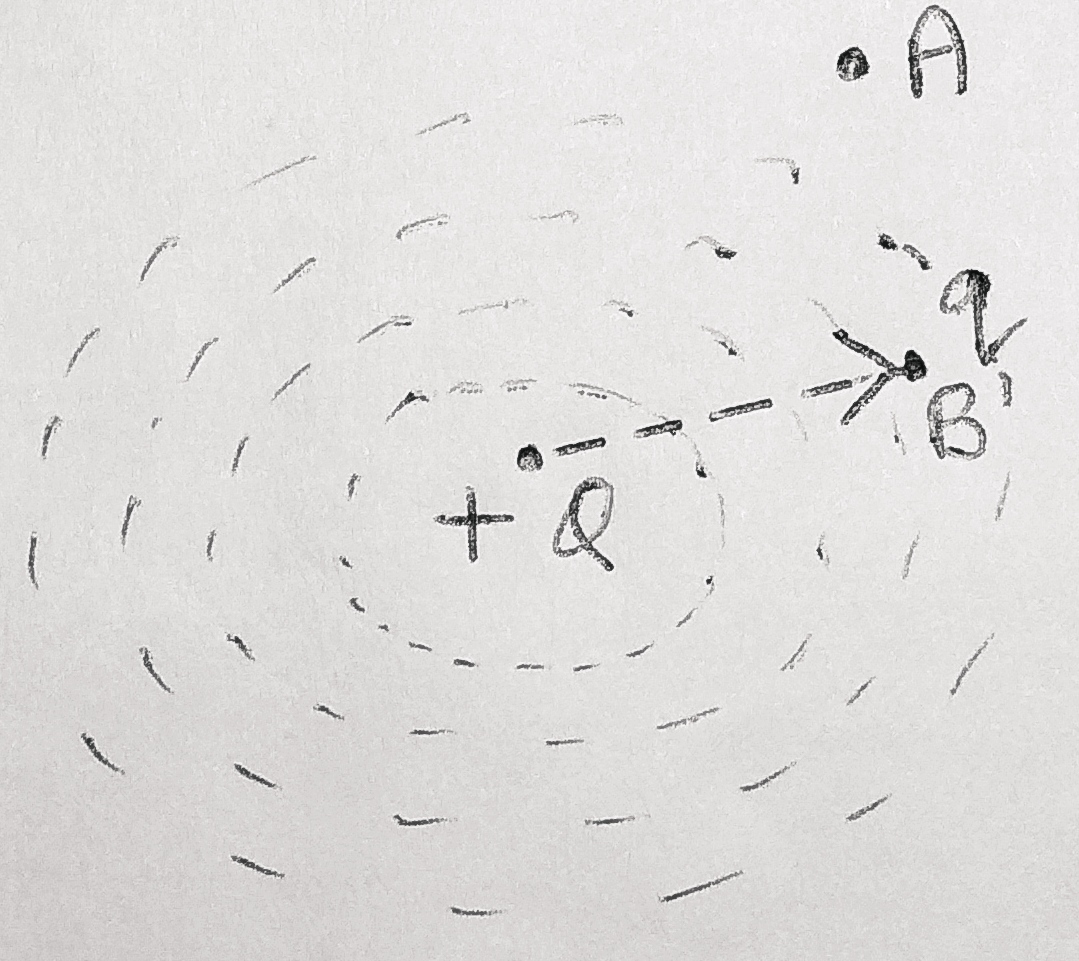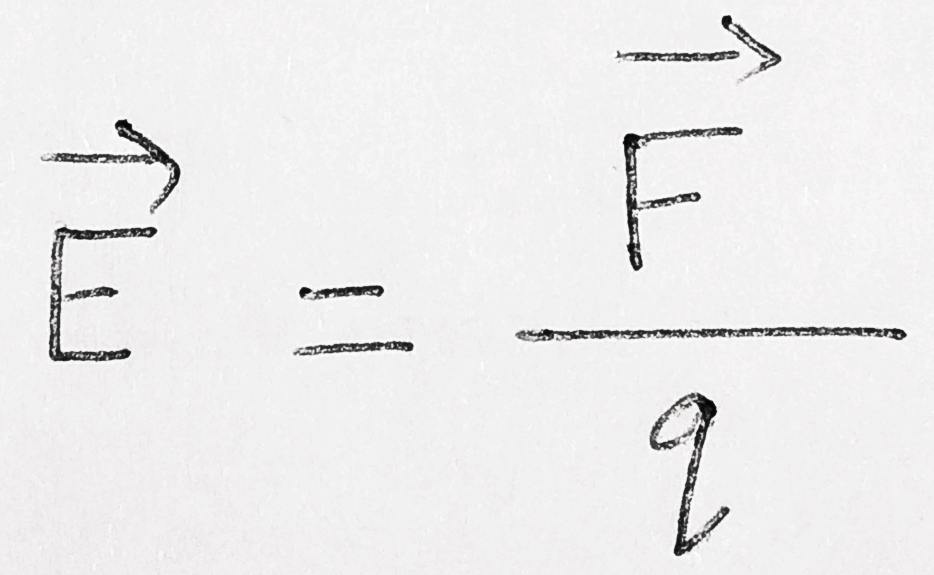# Electric Field & Electric Field Intensity

## Electric Field :

Electric field due to a given charge is defined as the region or space around the charge in which electrostatic force of attraction or repulsion due to the charge can be experienced by any other charge.Fig : imaginary region

(CONCEPT: in the above figure there is a charge +Q whose electric field is to be found. We consider two imaginary point A and B as shown in the figure, such that when a small charge +q is placed at point  A it does not experience any effect due to the charge Q, while at point B when it is placed it experiences a force of repulsion due to the charge Q.

In this situation we say that point A is outside the region of charge Q while the point B is inside the region of charge Q. Hence the electric field due to the charge Q is zero at point A and q will not experience any force due to Q at A.)

### Source Charge & Test Charge :

In above explanation the charge ' Q ' which is producing the electric field is called a ' Source Charge ' and the charge    ' q ' which is used to test the effect of source charge is called a ' Test Charge '.

### Electric Field Intensity

The electric field intensity at any point is defined as the force experienced by a unit positive charge placed at that point. It simply shows the strength of electric field at any point.

let us consider an electric field and a charge q is placed at any point in that field as shown in fig.In this case the electric field intensity at that points at charge q is given bywhere E is the electric field intensity and F is electrostatic force. The arrow sign above E and F  indicate that these are vector quantities.

SI Unit : N / C. ( Newton / coulomb )

Direction: Electric field intensity is a vector quantity and its direction  is same as direction of the force experienced by the charge at that point.